# Selina Solutions Concise Mathematics Class 6 Chapter 16 Percent (Percentage) Exercise 16(C)

Selina Solutions Concise Mathematics Class 6 Chapter 16 Percent (Percentage) Exercise 16(C) explains the steps to be followed in determining the percentage for the exercise wise problems. The solution PDF has detailed answers, according to the latest ICSE exam guidelines. These solutions also help students to analyze their weaknesses and work on them for better understanding. Students, who aim to improve problem solving skills, can use solutions PDF, designed by subject matter experts. In order to obtain a better hold on the concepts, students can download the Selina Solutions Concise Mathematics Class 6 Chapter 16 Percent (Percentage) Exercise 16(C) PDF, from the links which are provided below.

## Selina Solutions Concise Mathematics Class 6 Chapter 16: Percent (Percentage) Exercise 16(C) Download PDF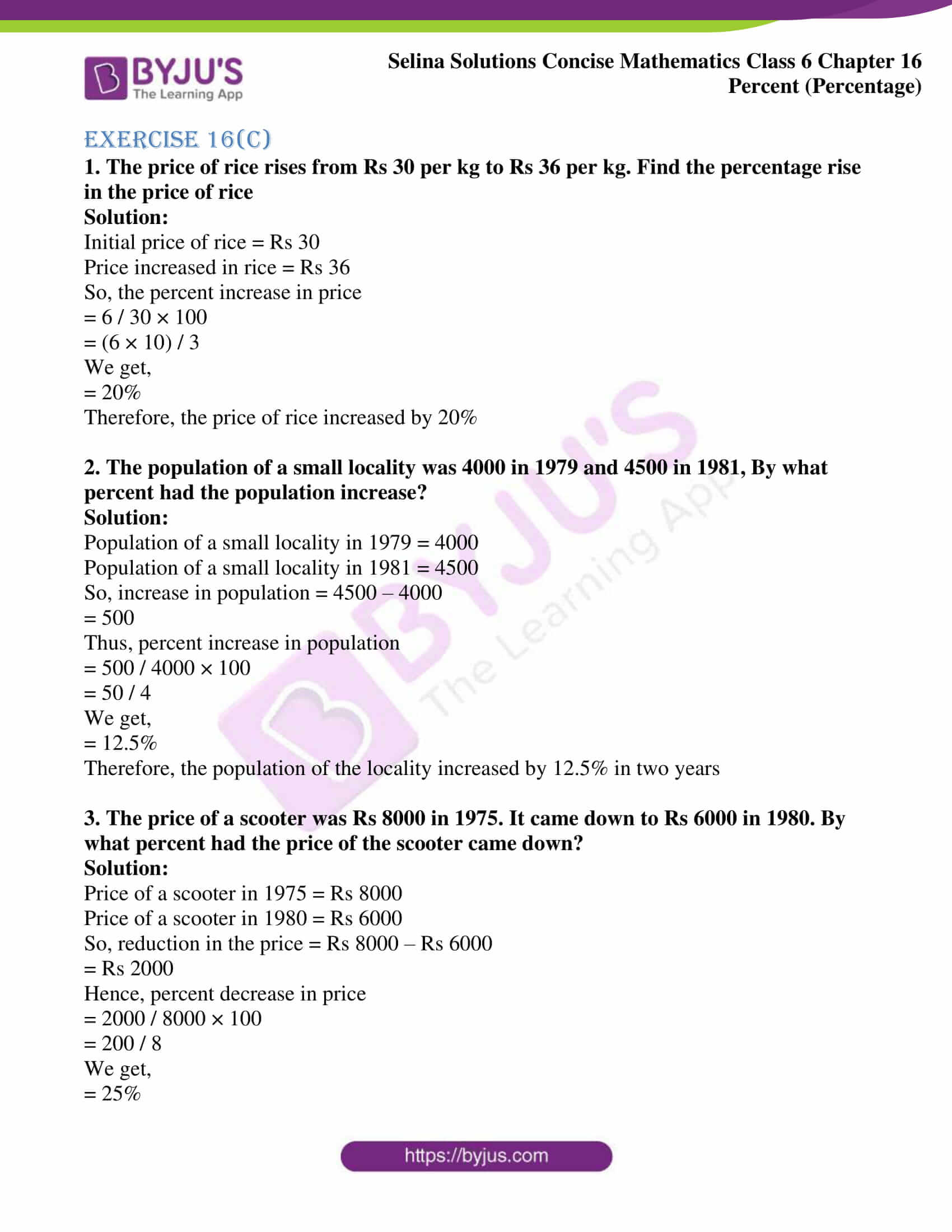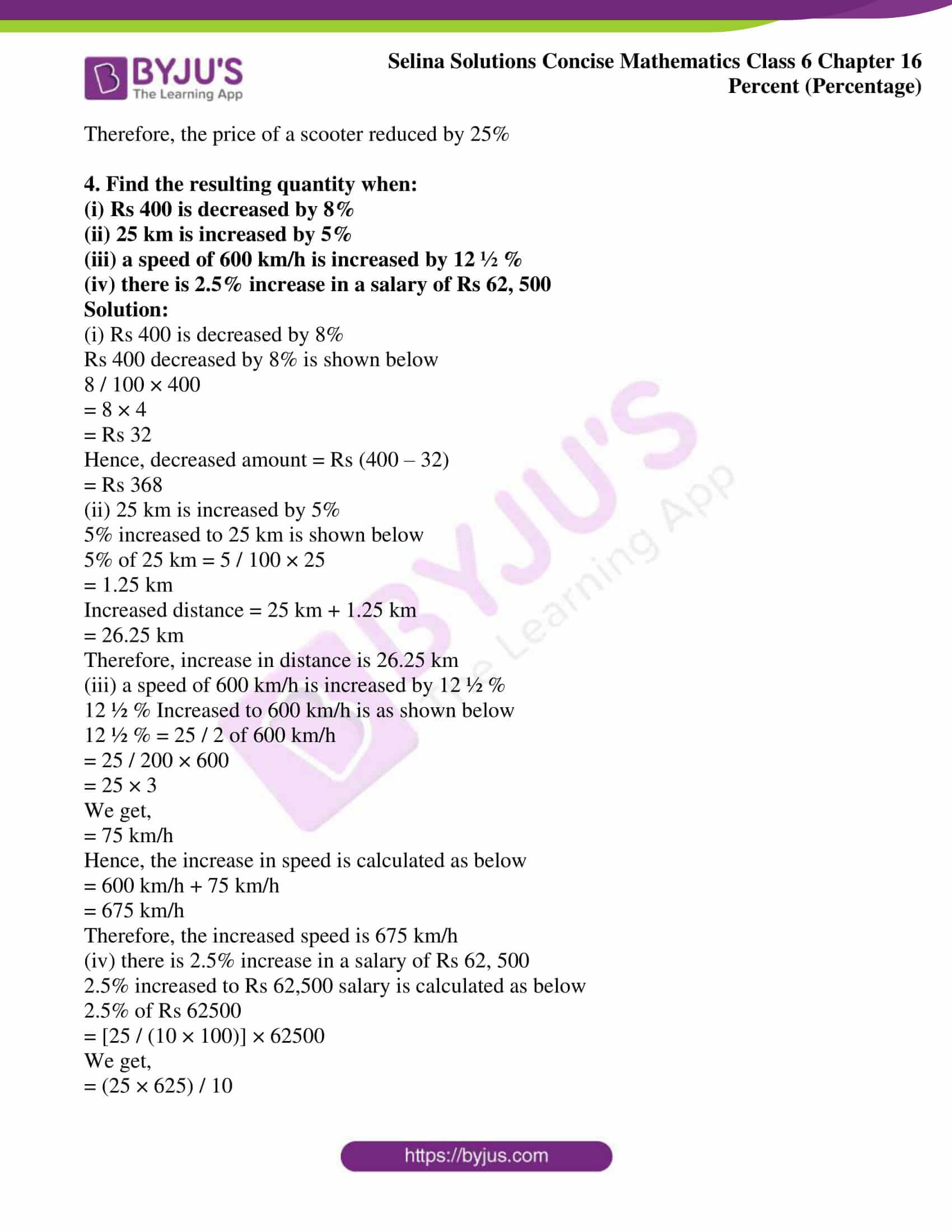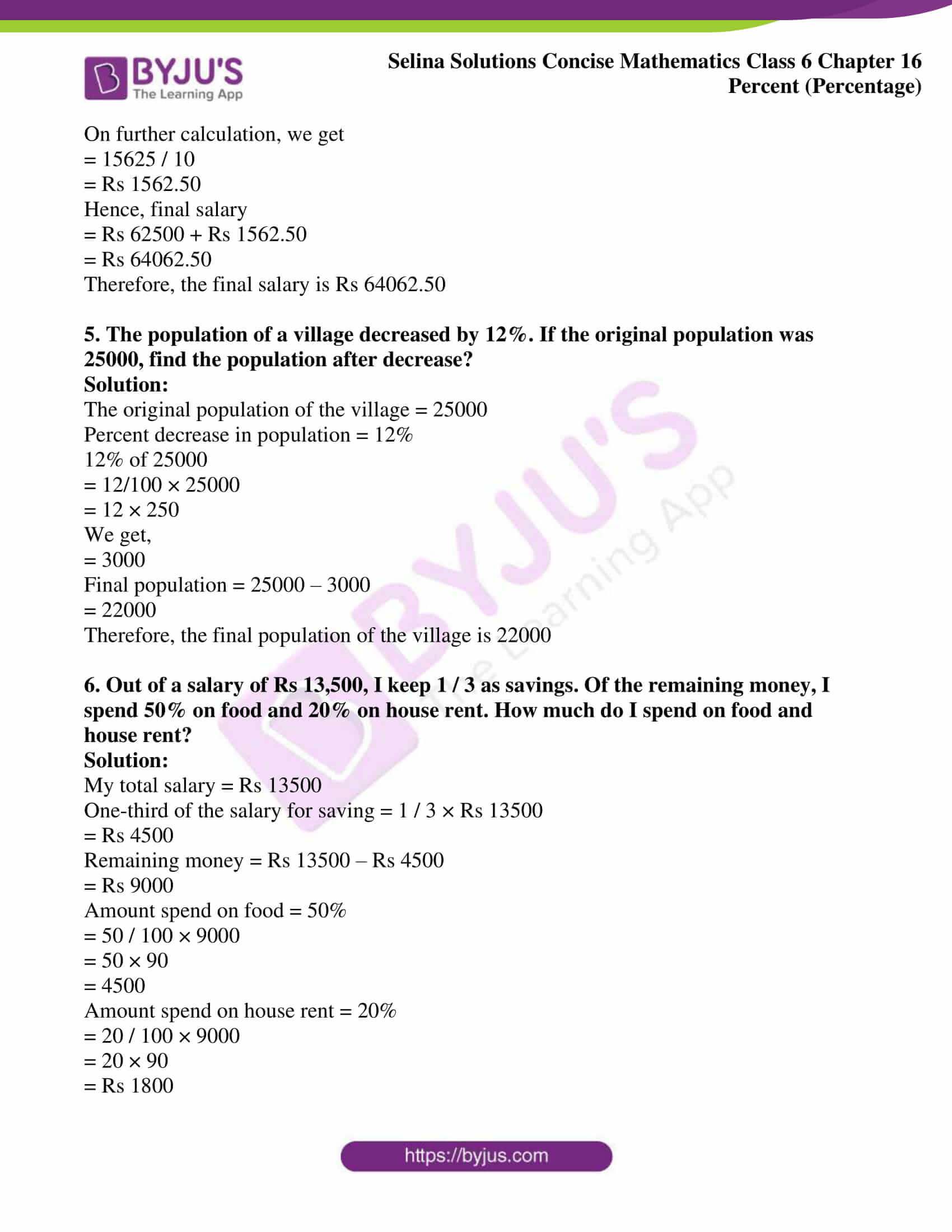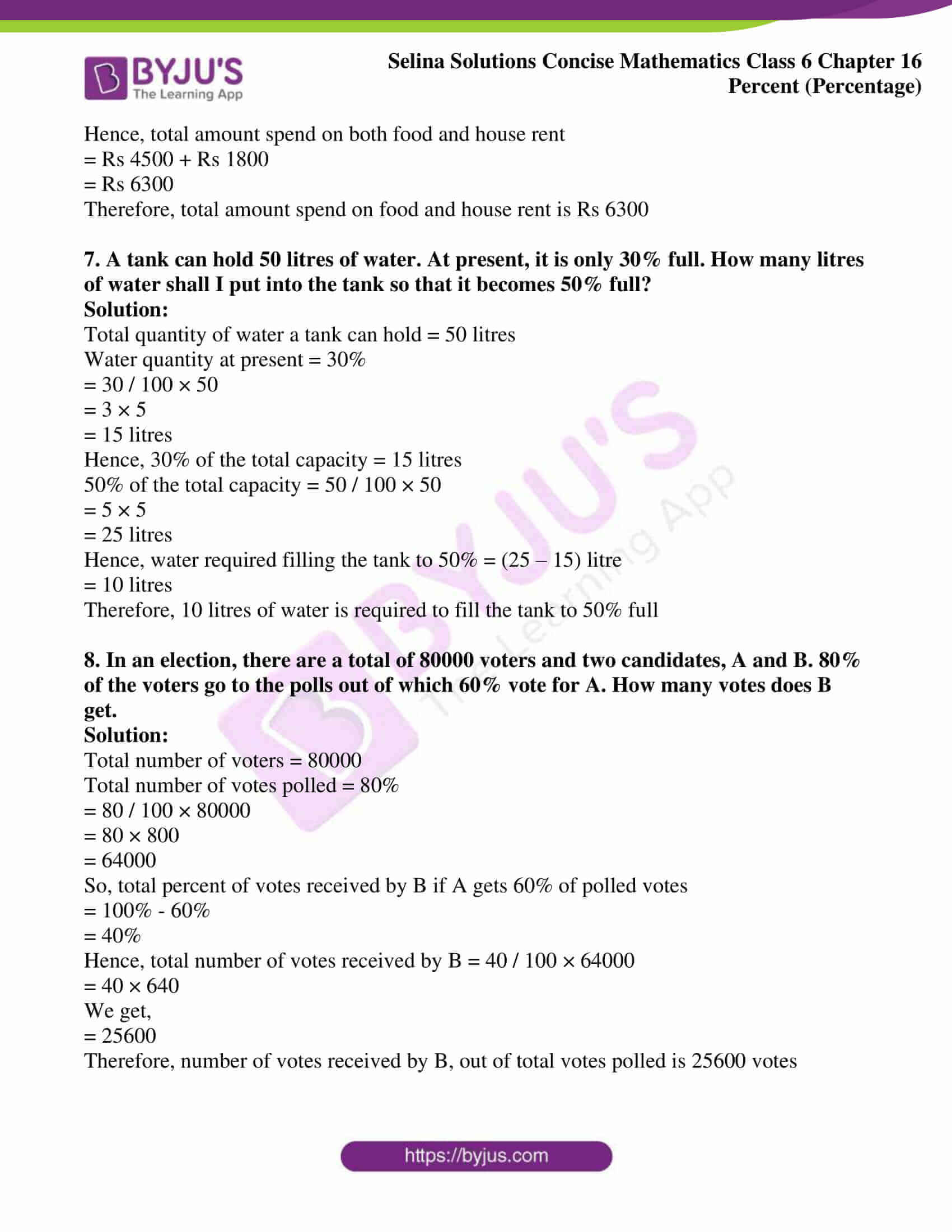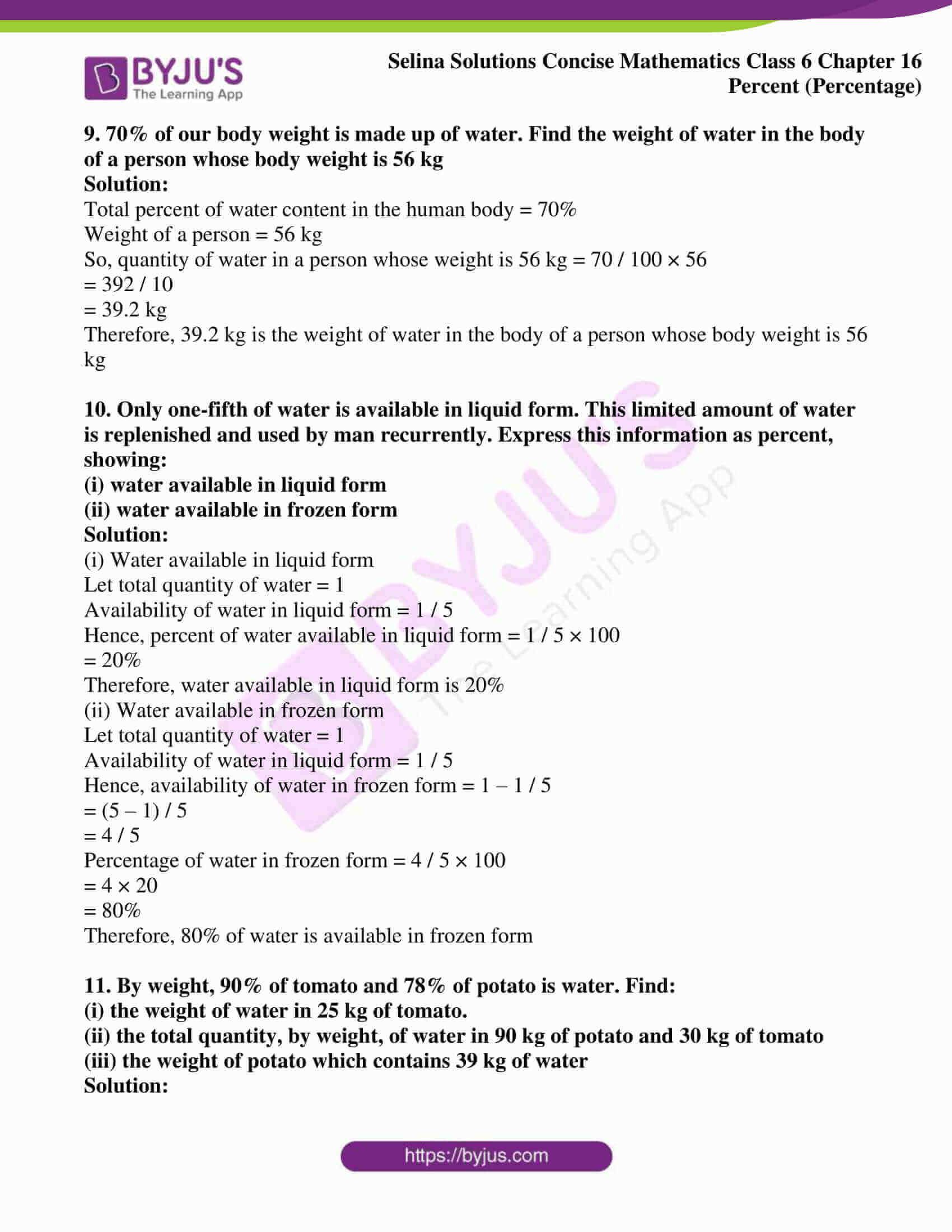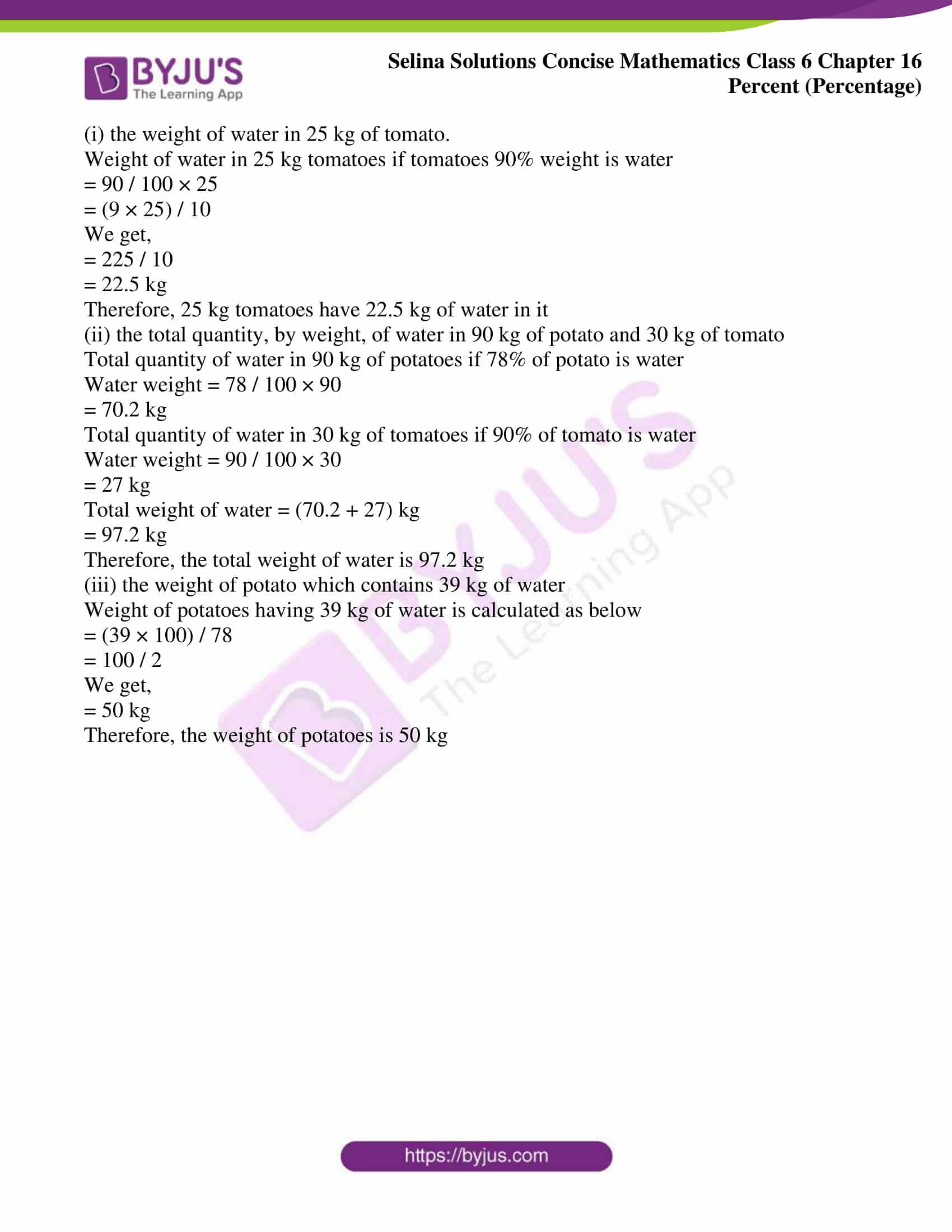### Access other exercises of Selina Solutions Concise Mathematics Class 6 Chapter 16: Percent (Percentage)

Exercise 16(A) Solutions

Exercise 16(B) Solutions

### Access Selina Solutions Concise Mathematics Class 6 Chapter 16: Percent (Percentage) Exercise 16(C)

1. The price of rice rises from Rs 30 per kg to Rs 36 per kg. Find the percentage rise in the price of rice

Solution:

Initial price of rice = Rs 30

Price increased in rice = Rs 36

So, the percent increase in price

= 6 / 30 × 100

= (6 × 10) / 3

We get,

= 20%

Therefore, the price of rice increased by 20%

2. The population of a small locality was 4000 in 1979 and 4500 in 1981, By what percent had the population increase?

Solution:

Population of a small locality in 1979 = 4000

Population of a small locality in 1981 = 4500

So, increase in population = 4500 – 4000

= 500

Thus, percent increase in population

= 500 / 4000 × 100

= 50 / 4

We get,

= 12.5%

Therefore, the population of the locality increased by 12.5% in two years

3. The price of a scooter was Rs 8000 in 1975. It came down to Rs 6000 in 1980. By what percent had the price of the scooter came down?

Solution:

Price of a scooter in 1975 = Rs 8000

Price of a scooter in 1980 = Rs 6000

So, reduction in the price = Rs 8000 – Rs 6000

= Rs 2000

Hence, percent decrease in price

= 2000 / 8000 × 100

= 200 / 8

We get,

= 25%

Therefore, the price of a scooter reduced by 25%

4. Find the resulting quantity when:

(i) Rs 400 is decreased by 8%

(ii) 25 km is increased by 5%

(iii) a speed of 600 km/h is increased by 12 ½ %

(iv) there is 2.5% increase in a salary of Rs 62, 500

Solution:

(i) Rs 400 is decreased by 8%

Rs 400 decreased by 8% is shown below

8 / 100 × 400

= 8 × 4

= Rs 32

Hence, decreased amount = Rs (400 – 32)

= Rs 368

(ii) 25 km is increased by 5%

5% increased to 25 km is shown below

5% of 25 km = 5 / 100 × 25

= 1.25 km

Increased distance = 25 km + 1.25 km

= 26.25 km

Therefore, increase in distance is 26.25 km

(iii) a speed of 600 km/h is increased by 12 ½ %

12 ½ % Increased to 600 km/h is as shown below

12 ½ % = 25 / 2 of 600 km/h

= 25 / 200 × 600

= 25 × 3

We get,

= 75 km/h

Hence, the increase in speed is calculated as below

= 600 km/h + 75 km/h

= 675 km/h

Therefore, the increased speed is 675 km/h

(iv) there is 2.5% increase in a salary of Rs 62, 500

2.5% increased to Rs 62,500 salary is calculated as below

2.5% of Rs 62500

= [25 / (10 × 100)] × 62500

We get,

= (25 × 625) / 10

On further calculation, we get

= 15625 / 10

= Rs 1562.50

Hence, final salary

= Rs 62500 + Rs 1562.50

= Rs 64062.50

Therefore, the final salary is Rs 64062.50

5. The population of a village decreased by 12%. If the original population was 25000, find the population after decrease?

Solution:

The original population of the village = 25000

Percent decrease in population = 12%

12% of 25000

= 12/100 × 25000

= 12 × 250

We get,

= 3000

Final population = 25000 – 3000

= 22000

Therefore, the final population of the village is 22000

6. Out of a salary of Rs 13,500, I keep 1 / 3 as savings. Of the remaining money, I spend 50% on food and 20% on house rent. How much do I spend on food and house rent?

Solution:

My total salary = Rs 13500

One-third of the salary for saving = 1 / 3 × Rs 13500

= Rs 4500

Remaining money = Rs 13500 – Rs 4500

= Rs 9000

Amount spend on food = 50%

= 50 / 100 × 9000

= 50 × 90

= 4500

Amount spend on house rent = 20%

= 20 / 100 × 9000

= 20 × 90

= Rs 1800

Hence, total amount spend on both food and house rent

= Rs 4500 + Rs 1800

= Rs 6300

Therefore, total amount spend on food and house rent is Rs 6300

7. A tank can hold 50 litres of water. At present, it is only 30% full. How many litres of water shall I put into the tank so that it becomes 50% full?

Solution:

Total quantity of water a tank can hold = 50 litres

Water quantity at present = 30%

= 30 / 100 × 50

= 3 × 5

= 15 litres

Hence, 30% of the total capacity = 15 litres

50% of the total capacity = 50 / 100 × 50

= 5 × 5

= 25 litres

Hence, water required filling the tank to 50% = (25 – 15) litre

= 10 litres

Therefore, 10 litres of water is required to fill the tank to 50% full

8. In an election, there are a total of 80000 voters and two candidates, A and B. 80% of the voters go to the polls out of which 60% vote for A. How many votes does B get.

Solution:

Total number of voters = 80000

Total number of votes polled = 80%

= 80 / 100 × 80000

= 80 × 800

= 64000

= 100% – 60%

= 40%

Hence, total number of votes received by B = 40 / 100 × 64000

= 40 × 640

We get,

= 25600

9. 70% of our body weight is made up of water. Find the weight of water in the body of a person whose body weight is 56 kg

Solution:

Total percent of water content in the human body = 70%

Weight of a person = 56 kg

So, quantity of water in a person whose weight is 56 kg = 70 / 100 × 56

= 392 / 10

= 39.2 kg

Therefore, 39.2 kg is the weight of water in the body of a person whose body weight is 56 kg

10. Only one-fifth of water is available in liquid form. This limited amount of water is replenished and used by man recurrently. Express this information as percent, showing:

(i) water available in liquid form

(ii) water available in frozen form

Solution:

(i) Water available in liquid form

Let total quantity of water = 1

Availability of water in liquid form = 1 / 5

Hence, percent of water available in liquid form = 1 / 5 × 100

= 20%

Therefore, water available in liquid form is 20%

(ii) Water available in frozen form

Let total quantity of water = 1

Availability of water in liquid form = 1 / 5

Hence, availability of water in frozen form = 1 – 1 / 5

= (5 – 1) / 5

= 4 / 5

Percentage of water in frozen form = 4 / 5 × 100

= 4 × 20

= 80%

Therefore, 80% of water is available in frozen form

11. By weight, 90% of tomato and 78% of potato is water. Find:

(i) the weight of water in 25 kg of tomato.

(ii) the total quantity, by weight, of water in 90 kg of potato and 30 kg of tomato

(iii) the weight of potato which contains 39 kg of water

Solution:

(i) the weight of water in 25 kg of tomato.

Weight of water in 25 kg tomatoes if tomatoes 90% weight is water

= 90 / 100 × 25

= (9 × 25) / 10

We get,

= 225 / 10

= 22.5 kg

Therefore, 25 kg tomatoes have 22.5 kg of water in it

(ii) the total quantity, by weight, of water in 90 kg of potato and 30 kg of tomato

Total quantity of water in 90 kg of potatoes if 78% of potato is water

Water weight = 78 / 100 × 90

= 70.2 kg

Total quantity of water in 30 kg of tomatoes if 90% of tomato is water

Water weight = 90 / 100 × 30

= 27 kg

Total weight of water = (70.2 + 27) kg

= 97.2 kg

Therefore, the total weight of water is 97.2 kg

(iii) the weight of potato which contains 39 kg of water

Weight of potatoes having 39 kg of water is calculated as below

= (39 × 100) / 78

= 100 / 2

We get,

= 50 kg

Therefore, the weight of potatoes is 50 kg